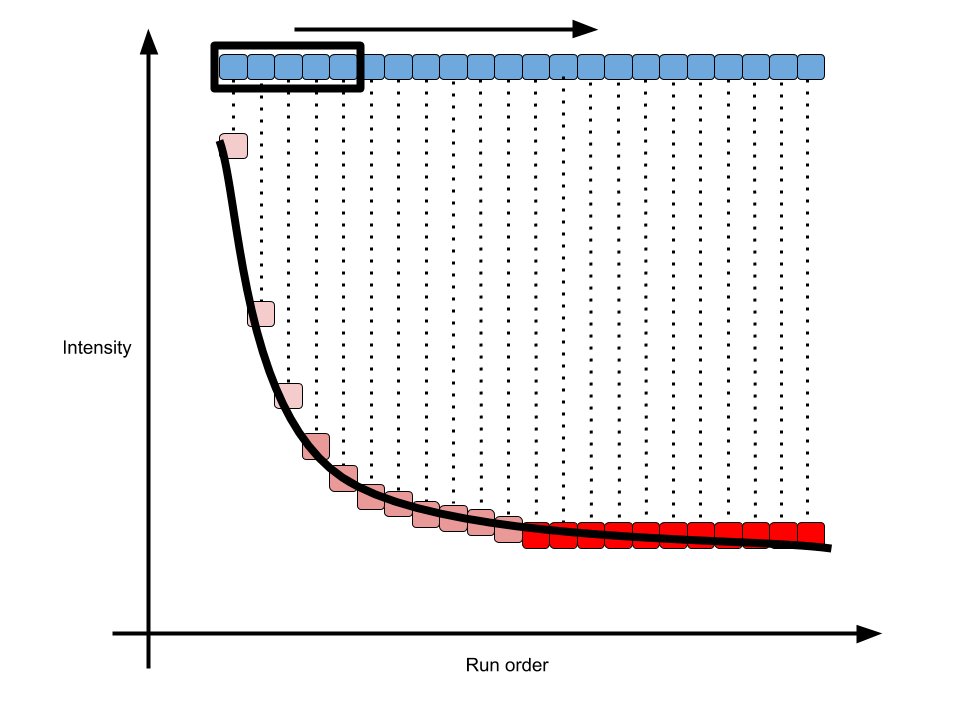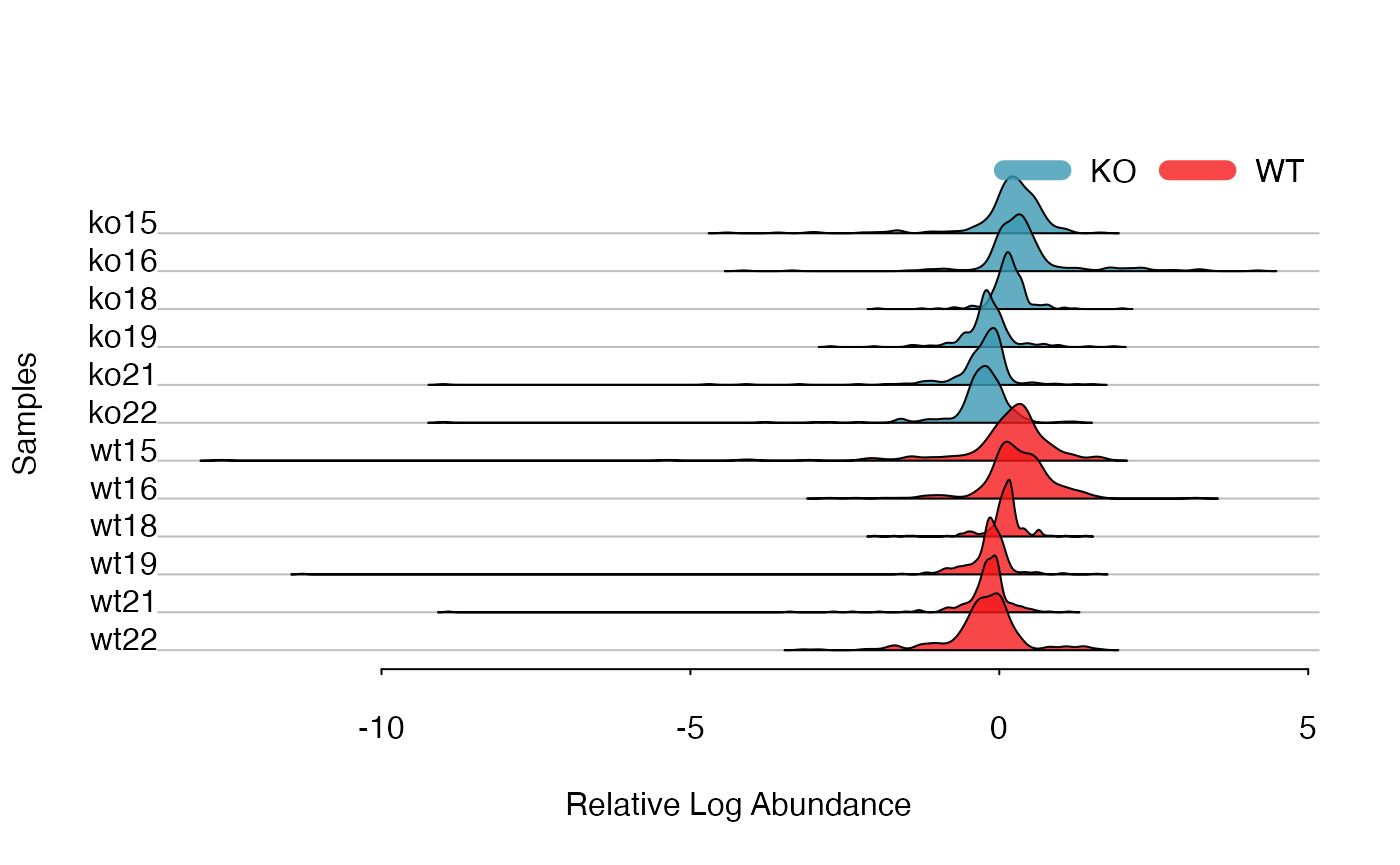# Run order effect for metabolomics studies

For a regular XC-MS based metabolomics workflow, the injection sequence should be carefully designed. Chromatograph column will always change and the first and last samples would show a shift of baseline. Such shifts would be monotone increasing or decreasing. In this case, we need some pooled QC samples to dirty the column at the very beginning of sequence. However, how many pooled QC samples will give us a stable baseline?

To solve this issue, I defined a Pooled QC Stable Index(PQSI) in my enviGCMS package. Instead of checking the TIC of one sample, I will check the stability of each peak one by one. Here is a demo:As shown in above figure, for one peak repeated analyzed in one sequence, the intensity would become stable in long term. In math, the slope of every n(5 is the default number) samples along the run order would become 0. Then we could define the percentage of stable peaks as PQSI. Such index would be a value between 0 and 1. The higher of such index, more peaks within the QC would be affected by run order effect. You could use such function to check the QC samples to see if run order effects would influence the samples at the beginning of sequences. I include this getpqsi function in enviGCMS package and here is the demo:

library(enviGCMS)
data(list)
order <- 1:12
# n means how many points to build a linear regression model
n = 5
idx <- getpqsi(list\$data,order,n = n)
plot(idx~order[-(1:(n-1))],pch=19)In this case, we could see at 5th sample, 30% peaks show correlation with the run order. However, ever since 6th sample, the run order effects could be ignore.